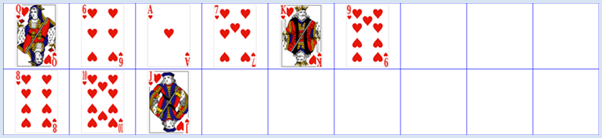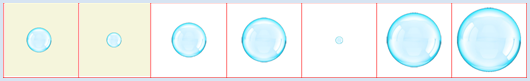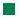deutsch     english    français     Print

## 10.1 COMPLEXITY WITH SORTING### INTRODUCTION

Computers are far more than pure calculating machines for simply crunching numbers. Rather, it is known that a considerable portion of the processing time of all computers active worldwide is used for sorting and searching data. It is therefore important that a program not only provides the correct data, but is also optimized. This applies to:

• its length
• its structure and clarity
• its execution time
• its memory usage

Basically, one should already think of optimizing in the beginning of the development process, since it is usually difficult to optimize a sloppily written program afterwards.

In this chapter you will examine the optimization of the execution time when sorting data. You will also get to know the limits of computer science and computer use because a problem, for which there is probably an algorithmic solution process but even the fastest computer would need hundreds of years to solve, is considered to be an unsolvable problem.

 PROGRAMMING CONCEPTS: Complexity, execution time, order of algorithms, sorting methods, overloading operators

### SORTING LIKE CHILDREN: CHILDREN SORT

 The sorting and ordering of a set of objects with the comparative operations larger, smaller and equal [more... In mathematics this is called an order relation] is and remains a standard task in computer science. Although you will find library routines in all common high-level programming languages that you can sort with, you should include the concepts of sorting to your standard knowledge because there are always situations where you need to implement or optimize the sorting yourself. A collection of unsorted objects is referred to as a set. The objects are saved in a one-dimensional data structure in the computer where a list is especially well suited [more... Earlier arrays were often used, but have the disadvantage of a fixed length]. In the program, consider the dwarfs to be actors of the game library JGameGrid. You can easily display their sprite images in a grid [more... With today's programming languages it is possible without significant additional effort to sort interesting objects as numbers]. The height of the sprite image (in pixels) also serves as a measure of the body size.Algorithms are often borrowed directly from processes that we also apply in your everyday life. If you ask children how they organize a set of objects by size, they often describe the process as follows: "You take the smallest (or biggest) object and set it down in order". This solution process seems very plausible, but there is a problem for the computer because it can not determine the smallest or biggest object like humans can with just a simple glance. It must first look for the smallest object in the unordered list by running through all the objects in order and comparing them. To implement the sorting process, in this case called children sort, you need a function getSmallest(row) which returns the smallest dwarf from the reviewed list. You can start as follows: Save the first list element in the variable smallest and run through all subsequent elements in a for-loop. If the element you are looking at is smaller than smallest, replace smallest with it. You use two lists for children sort, one list startList with the given objects and another list targetList which is initially empty. You search for the smallest element in startList, remove it from there, and then add it to the back of targetList until startList is empty. ```from gamegrid import * from random import shuffle def bodyHeight(dwarf): return dwarf.getImage().getHeight() def updateGrid(): removeAllActors() for i in range(len(startList)): addActor(startList[i], Location(i, 0)) for i in range(len(targetList)): addActor(targetList[i], Location(i, 1)) def getSmallest(li): global count smallest = li for dwarf in li: count += 1 if bodyHeight(dwarf) < bodyHeight(smallest): smallest = dwarf return smallest n = 7 makeGameGrid(n, 2, 170, Color.red, False) setBgColor(Color.white) show() startList = [] targetList = [] for i in range(0 , n): dwarf = Actor("sprites/dwarf" + str(i) + ".png") startList.append(dwarf) shuffle(startList) updateGrid() setTitle("Children Sort. Press to sort...") count = 0 while not isDisposed() and len(startList) > 0: c = getKeyCodeWait() if c == 32: smallest = getSmallest(startList) targetList.append(smallest) startList.remove(smallest) count += 1 setTitle("Count: " + str(count) + " for next step...") updateGrid() setTitle("Count: " + str(count) + " All done") ``` Highlight program code (Ctrl+C copy, Ctrl+V paste)

### MEMO

With children sort, besides the given unordered list of length n, you need a second list that eventually also has the length n. If n is very large this could lead to a memory problem. [more... Sorting algorithms, which require a second data structure of the same length, are called
out-of-place, or ex situ. They are seldom used because of the need for additional storage space
].

You can easily figure out how many elementary steps are required to solve the problem: Regardless of how the objects are arranged in the given list, you first need to run through all n elements of the list, then through n-1, etc. In addition to this, you have to perform the operation of moving the found element from the start list to the target list each time. The number of operations c is therefore the sum of all natural numbers from 2 to n + 1 as you can see with your variable count. Using the formula for sums of natural numbers we get:

 c = ((n + 1)*(n + 2))/         2 - 1 = (n2)/ 2 - (3n)/ 2

For example, when n = 1000 we already get

 c = 1000*1000/         2 + 3*1000/    2 = 500000 + 1500 ≈ 500000

steps! As you can see, the quadratic term prevails for large n values and this is why we say
The complexity of the algorithm is of the n-square order
which we write as:

Complexity = O(n2)

### SORTING THE CARD GAME: INSERTION SORT

 When you hold the cards in a fan shape while playing cards, you often intuitively use another sorting method: You add each newly obtained card to your 'fan' in a particular place where it fits best according to its value. In your program that inserts the disordered cards from the start list (the deck) into the target list (your hand), you proceed exactly in this way: You take card by card from left to right from the start list and run though the already ordered target list from the left to right as well. As soon as the card you picked up is considered to be higher in value than the last one considered in your hand, you add it to the target list right there.```from gamegrid import * from random import shuffle def cardValue(card): return card.getImage().getHeight() def updateGrid(): removeAllActors() for i in range(len(startList)): addActor(startList[i], Location(i, 0)) for i in range(len(targetList)): addActor(targetList[i], Location(i, 1)) n = 9 makeGameGrid(n, 2, 130, Color.blue, False) setBgColor(Color.white) show() startList = [] targetList = [] for i in range(0 , 9): card = Actor("sprites/" + "hearts" + str(i) + ".png") startList.append(card) shuffle(startList) updateGrid() setTitle("Insertion Sort. Press to sort...") count = 0 while not isDisposed() and len(startList) > 0: getBg().clear() c = getKeyCodeWait() if c == 32: pick = startList # take first startList.remove(pick) i = 0 while i < len(targetList) and cardValue(pick) > cardValue(targetList[i]): i += 1 count += 1 targetList.insert(i, pick) count += 1 setTitle("Count: " + str(count) + " for next step...") updateGrid() setTitle("Count: " + str(count) + " All done") ``` Highlight program code (Ctrl+C copy, Ctrl+V paste)

### MEMO

 This sorting method is called insertion sort. The number of steps necessary depends on the order of the cards in the deck. The most steps are needed in a situation where the deck of cards is coincidentally ordered in reverse. One can reflect about it, or find out with a computer simulation, that the number of steps on average (for large n) increase with n2 / 4 , and thus the average complexity is also O(n2), as with children sort.

### SORTING WITH BUBBLES: BUBBLE SORT

 A known way to sort objects in a list is to repeatedly run through the list from left to right and to always swap two adjacent elements if they are in the wrong order. With this method, first the largest element moves successively from left to right until it has arrived in the final cell. In the next pass you start again on the left, but only go up to the second to last element since the largest is already in place. No second list is necessary in this process [more... We call such a sorting process in-place or in-situ].```from gamegrid import * from random import shuffle def bubbleSize(bubble): return bubble.getImage().getHeight() def updateGrid(): removeAllActors() for i in range(len(li)): addActor(li[i], Location(i, 0)) def exchange(i, j): temp = li[i] li[i] = li[j] li[j] = temp n = 7 li = [] makeGameGrid(n, 1, 150, Color.red, False) setBgColor(Color.white) show() for i in range(0 , n): bubble = Actor("sprites/bubble" + str(i) + ".png") li.append(bubble) shuffle(li) updateGrid() setTitle("Bubble Sort. Press for next step...") k = n - 1 i = 0 count = 0 while not isDisposed() and k > 0: getBg().fillCell(Location(i, 0), makeColor("beige")) getBg().fillCell(Location(i + 1, 0), makeColor("beige")) refresh() c = getKeyCodeWait() if c == 32: count += 1 bubble1 = li[i] bubble2 = li[i + 1] refresh() if bubbleSize(bubble1) > bubbleSize(bubble2): exchange(i, i + 1) setTitle("Last Action: Exchange. Count: " + str(count)) else: setTitle("Last Action: No Exchange. Count: " + str(count)) getBg().clear() updateGrid() if i == k - 1: k = k - 1 i = 0 else: i += 1 getBg().clear() refresh() setTitle("All done. Count: " + str(count)) ``` Highlight program code (Ctrl+C copy, Ctrl+V paste)

### MEMO

 The largest elements move over to the right, just like bubbles move up in water. Because of this, the name of this sorting algorithm is bubble sort. You can think about it, or check the built-in step counter, its complexity is independent of the arrangement of the elements in the specified list, but again of the order O(n2). To make the demonstration a bit more exciting, both cells whose bubbles were compared last are colored using the background method fillCell(). The background color can be cleared with getBg().clear(). You have to call refresh() so that the image is re-rendered on the screen.

### SORTING WITH LIBRARY ROUTINES: TIMSORT

 Since sorting is one of the most important tasks, all high-level programming languages provide built-in library functions for sorting. In Python, the function sorted(list, cmp) even belongs to the standard built-in functions, which means that it can be used without having to import anything. You can thus save yourself from having to write a sorting algorithm, but in order to do that you have to learn how the library function is used. Clearly it need the list to be sorted as a parameter. With a second parameter, you have to tell it according to which classification criterion it should sort the objects. You define the sorting criterion in a function which here you call compare(). This function has to obtain two objects as parameters and return one of the three values 1, 0, and -1, depending on whether the first object is greater, equal to, or less than the second object. You pass the library function sorted() your freely chosen function name as a second parameter or using the named parameter cmp. ```from gamegrid import * from random import shuffle def bodyHeight(dwarf): return dwarf.getImage().getHeight() def compare(dwarf1, dwarf2): if bodyHeight(dwarf1) < bodyHeight(dwarf2): return -1 elif bodyHeight(dwarf1) > bodyHeight(dwarf2): return 1 else: return 0 def updateGrid(): removeAllActors() for i in range(len(li)): addActor(li[i], Location(i, 0)) n = 7 li = [] makeGameGrid(n, 1, 170, Color.red, False) setBgColor(Color.white) show() for i in range(0 , n): dwarf = Actor("sprites/dwarf" + str(i) + ".png") li.append(dwarf) shuffle(li) updateGrid() setTitle("Timsort. Press any key to get result...") getKeyCodeWait() li = sorted(li, cmp = compare) updateGrid() setTitle("All done.") ``` Highlight program code (Ctrl+C copy, Ctrl+V paste)

### MEMO

 If you want to use library functions to sort, you have to specify with a comparison function how two elements are compared to find out whether they are greater, equal or lesser [more... For a list of numbers that you'd sort ascending, you need no comparison indicate, the usual number automatically comparison is used. For a list of strings the alphabetic comparison is used]. The algorithm used in Python was invented by Tim Peters in 2002 and is thus called Timsort. It has (on average) the order O(nlog(n)). So, for example, when n = 106 only about 107 operations are necessary instead of about 1012 as it would be with a sorting algorithm with the order O(n2).

### EXERCISES

 1 Sort the 7 dwarfs with a bubble sort. 2 Add the sprite image snowwhite.png of Snow White that has the same size as the largest dwarf into the bubble sort from exercise 1. Show that the order of Snow White and the largest dwarf always correspond to their order in the start list. (Such a sorting algorithm is called stable.) 3 You can very easily generate long unsorted lists of numbers using row = range(n) and subsequently random.shuffle(row). Measure the execution time of the internal sorting algorithm (Timsort) for different values of n and show that the complexity is much better than O(n2). Instructions: In order to measure a time difference, import the module time and calculate the difference between two calls of time.clock().

###Comparing two objects is an important operation. You can use the 5 comparison operations <, <=, ==, >, = > for numbers. In Python it is possible to apply these operators to some other data types, for example for dwarfs. Through this, your code gains elegance and clarity. You can do the following: In the class definition of your data type, define the methods _lt_(), __le__(), __eq__(), __ge__(), __gt__() that return the Boolean values of the comparison operations less, less-and-equal, equal, greater-and-equal, greater. In addition, you can also define the method __str()__, which is used when the function str() is called. However this has nothing to do with sorting. In the class Dwarf (derived from Actor), you also save the name of the dwarf as an instance variable that you can write out as a TextActor upon updateGrid().```from gamegrid import * from random import shuffle class Dwarf(Actor): def __init__(self, name, size): Actor.__init__(self, "sprites/dwarf" + str(size) + ".png") self.name = name self.size = size def __eq__(self, a): # == return self.size == a.size def __ne__(self, a): # != return self.size != a.size def __gt__(self, a): # > return self.size > a.size def __lt__(self, a): # < return self.size < a.size def __ge__(self, a): # >= return self.size >= a.size def __le__(self, a): # <= return self.size <= a.size def __str__(self): # str() function return self.name def compare(dwarf1, dwarf2): if dwarf1 < dwarf2: return -1 elif dwarf1 > dwarf2: return 1 else: return 0 def updateGrid(): removeAllActors() for i in range(len(row)): addActor(row[i], Location(i, 0)) addActor(TextActor(str(row[i])), Location(i, 0)) n = 7 row = [] names = ["Monday", "Tuesday", "Wednesday", "Thursday", "Friday", "Saturday", "Sunday"] makeGameGrid(n, 1, 170, Color.red, False) setBgColor(Color.white) show() for i in range(0 , n): dwarf = Dwarf(names[i], i) row.append(dwarf) shuffle(row) updateGrid() setTitle("Press any key to get result...") getKeyCodeWait() row = sorted(row, cmp = compare) updateGrid() setTitle("All done.") ``` Highlight program code (Ctrl+C copy, Ctrl+V paste)

### MEMO

 The use of comparison operators for arbitrary data types is not mandatory, but elegant. One says that the operators are overloaded.